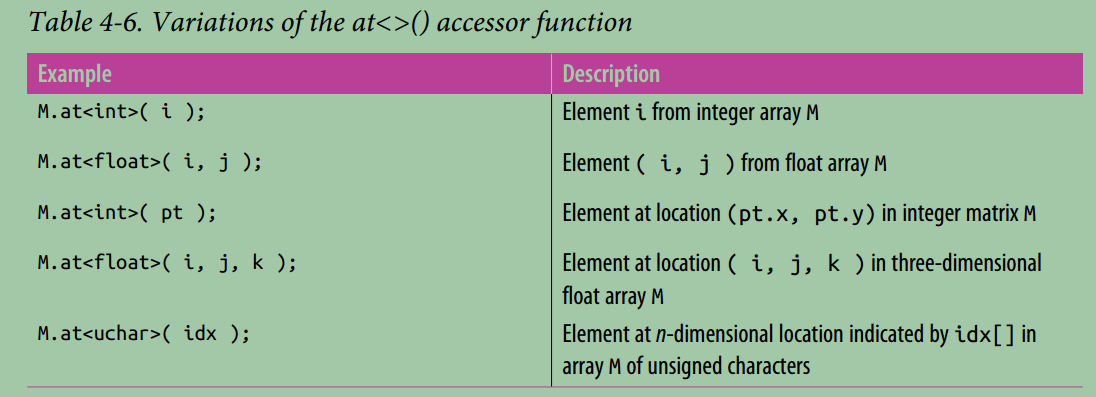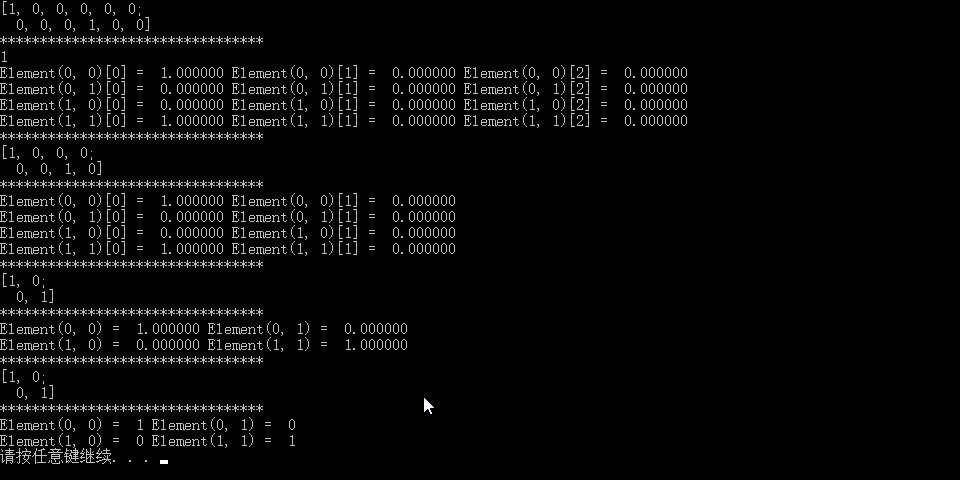learning opencv3: 四：Mat Accessing Array Elements Individually

2020/12/16 12:55

The basic means of direct access is the (template) member function at<>(). There are
many variations of this function that take different arguments for arrays of different
numbers of dimensions. The way this function works is that you specialize the
at<>() template to the type of data that the matrix contains, then access that element
using the row and column locations of the data you want. Here is a simple example:As with at<>(), ptr<>() is a template function instantiated with a type name. It takes an integer argument indicating the row you wish to get a pointer to.

The function returns a pointer to the primitive type of which the array is constructed (i.e., if the array type is CV_32FC3, the return value will be of type float*).

isContinuous()

There is one last important point to keep in mind about C-style pointer access. If you
want to access everything in an array, you will likely want to iterate one row at a time;
this is because the rows may or may not be packed continuously in the array. How‐
ever, the member function isContinuous() will tell you if the members are continu‐
ously packed. If they are, you can just grab the pointer to the very first element of the
first row and cruise through the entire array as if it were a giant one-dimensional array

#include <iostream>
#include <opencv2/imgproc/imgproc.hpp>
#include <opencv2/highgui/highgui.hpp>

using namespace cv;

int main(int argc, char** argv)
{

Mat oneMat = Mat::eye(2, 2, CV_32FC3);
std::cout << oneMat << std::endl;
std::cout << "*********************************" << std::endl;
std::cout << oneMat.isContinuous()  << std::endl;

for (size_t i = 0; i < 2; i++)
{
for (size_t j = 0; j < 2; j++)
{
for (size_t k = 0; k < 3; k++)
{
printf("Element(%d, %d)[%d] = % f ", i, j, k, oneMat.at<cv::Vec3f>(i, j)[k]);
}
printf("\n");
}
}
std::cout << "*********************************" << std::endl;

Mat oneMat2 = Mat::eye(2, 2, CV_32FC2);
std::cout << oneMat2 << std::endl;
std::cout << "*********************************" << std::endl;

for (size_t i = 0; i < 2; i++)
{
for (size_t j = 0; j < 2; j++)
{
for (size_t k = 0; k < 2; k++)
{
printf("Element(%d, %d)[%d] = % f ", i, j, k, oneMat2.at<cv::Vec2f>(i, j)[k]);
}
printf("\n");
}
}
std::cout << "*********************************" << std::endl;

Mat oneMat3 = Mat::eye(2, 2, CV_32FC1);
std::cout << oneMat3 << std::endl;
std::cout << "*********************************" << std::endl;

for (size_t i = 0; i < 2; i++)
{
for (size_t j = 0; j < 2; j++)
{
printf("Element(%d, %d) = % f ", i, j, oneMat3.at<float>(i, j));
}
printf("\n");

}
std::cout << "*********************************" << std::endl;

Mat oneMat4 = Mat::eye(2, 2, CV_8UC1);
std::cout << oneMat4 << std::endl;
std::cout << "*********************************" << std::endl;

for (size_t i = 0; i < 2; i++)
{
for (size_t j = 0; j < 2; j++)
{
printf("Element(%d, %d) = % d ", i, j, oneMat4.at<uchar>(i, j));
}
printf("\n");
}

getchar();
system("pause");

return 0;
}0
0 收藏

作者的其它热门文章0 评论
0 收藏
0